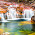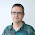## Monday, March 24, 2014

### Shaded relief images using GDAL python

After showing how to colour a DEM file, classifying it, and calculating its isobands, this post shows how to create a shaded relief image from it.The resulting image
A shaded relief image simulates the shadow thrown upon a relief map. This shadow is usually blended with some colouring, related to the altitude, a terrain classification, etc.
The shadow is usually drawn considering that the sun is at 315 degrees of azimuth and 45 degrees over the horizon, which never happens at the north hemisphere. This values avoid strange perceptions, such as seeing the mountain tops as the bottom of a valley.

In this example, the script calculates the hillshade image, a coloured image, and blends them into the shaded relief image.

As usual, all the code, plus the sample DEM file, can be found at GitHub.

I didn't know how to create a shaded relief image using numpy. Eric Gayer helped me with some samples, and I found some other information here.
The script is:
"""
Creates a shaded relief file from a DEM.
"""

from osgeo import gdal
from numpy import pi
from numpy import arctan
from numpy import arctan2
from numpy import sin
from numpy import cos
from numpy import sqrt
from numpy import zeros
from numpy import uint8
import matplotlib.pyplot as plt

slope = pi/2. - arctan(sqrt(x*x + y*y))
aspect = arctan2(-x, y)

ds = gdal.Open('w001001.tiff')
band = ds.GetRasterBand(1)

plt.imshow(hs_array,cmap='Greys')
plt.show()

• The script draws the image using matplotlib, to make it easy
• The hillshade function starts calculating the gradient for the x and y directions using the numpy.gradient function. The result are two matrices of the same size than the original, one for each direction.
• From the gradient, the aspect and slope can be calculated. The aspect will give the mountain orientation, which will be illuminated depending on the azimuth angle. The slopewill change the illumination depending on the altitude angle.
• Finally, the hillshade is calculated.

The shaded relief image is calculated using the algorithm explained in the post Colorize PNG from a raster file and the hillshade.
As in the coloring post, the image is read by blocks to improve the performance, because it uses a lot of arrays, and doing it at once with a big image can take a lot of resources.
I will coment the code block by block, to make it easier. The full code is here.

The main function, called shaded_relief, is the most important, and calls the different algorithms:
def shaded_relief(in_file, raster_band, color_file, out_file_name,
azimuth=315, angle_altitude=45):
'''
The main function. Reads the input image block by block to improve the performance, and calculates the shaded relief image
'''

if exists(in_file) is False:
raise Exception('[Errno 2] No such file or directory: \'' + in_file + '\'')

if dataset == None:
raise Exception("Unable to read the data file")

band = dataset.GetRasterBand(raster_band)

block_sizes = band.GetBlockSize()
x_block_size = block_sizes
y_block_size = block_sizes

#If the block y size is 1, as in a GeoTIFF image, the gradient can't be calculated,
#so more than one block is used. In this case, using8 lines gives a similar
#result as taking the whole array.
if y_block_size < 8:
y_block_size = 8

xsize = band.XSize
ysize = band.YSize

max_value = band.GetMaximum()
min_value = band.GetMinimum()

#Adding an extra value to avoid problems with the last & first entry
if sorted(color_table.keys()) > min_value:
color_table[min_value - 1] = color_table[sorted(color_table.keys())]

if sorted(color_table.keys())[-1] < max_value:
color_table[max_value + 1] = color_table[sorted(color_table.keys())[-1]]
#Preparing the color table
classification_values = color_table.keys()
classification_values.sort()

max_value = band.GetMaximum()
min_value = band.GetMinimum()

if max_value == None or min_value == None:
stats = band.GetStatistics(0, 1)
max_value = stats
min_value = stats

out_array = zeros((3, ysize, xsize), 'uint8')

#The iteration over the blocks starts here
for i in range(0, ysize, y_block_size):
if i + y_block_size < ysize:
rows = y_block_size
else:
rows = ysize - i

for j in range(0, xsize, x_block_size):
if j + x_block_size < xsize:
cols = x_block_size
else:
cols = xsize - j

dem_array = band.ReadAsArray(j, i, cols, rows)

angle_altitude)

rgb_array = values2rgba(dem_array, color_table,
classification_values, max_value, min_value)

hsv_array = rgb_to_hsv(rgb_array[:, :, 0],
rgb_array[:, :, 1], rgb_array[:, :, 2])

hsv_array, hs_array] )

#Saving the image using the PIL library
im = fromarray(transpose(out_array, (1,2,0)), mode='RGB')
im.save(out_file_name)
• After opening the file, at line 20 comes the first interesting point. If the image is read block by block, some times the blocks will have only one line, as in the GeoTIFF images. With this situation, the y gradient won't be calculated, so the hillshade function will fail. I've seen that taking only two lines gives coarse results, and with lines the result is more or less the same as taking the whole array. The performance won't be as good as using only one block, but works faster anyway.
• Lines 32 to 51 read the color table and file maximim and minumum. This has to be outside the values2rgba function, since is needed only once.
• Lines 54 to 66 control the block reading. For each iteration, a small array will be read (line 67). This is what will be processed. The result will be written in the output array defined at line 52, that has the final size.
• Now the calculations start:
• At line 69, the hillshade is calculated
• At line 72, the color array is calculated
• At line 75, the color array is changed from rgb values to hsv.
• At line 78, the value (the v in hsv) is changed to the hillshade value. This will blend both images. I took the idea from this post.
• Then the image is transformed to rgb again (line 81) and written into the output array (line 83)
• Finally, the array is transformed to a png image using the PIL library. The numpy.transpose function is used to re-order the matrix, since the original values are with the shape (3, height, width), and the Image.fromarray function needs (height, width, 3). An other way to do this is using scipy.misc.imsave (that would need scipy installed just for that), or the Image.merge function.

The colouring funcion is taken from the post  Colorize PNG from a raster file, but modifying it so the colors are only continuous, since the discrete option doesn't give nice results in this case:
def values2rgba(array, color_table, classification_values, max_value, min_value):
'''
This function calculates a the color of an array given a color table.
The color is interpolated from the color table values.
'''
rgba = zeros((array.shape, array.shape, 4), dtype = uint8)

for k in range(len(classification_values) - 1):
if classification_values[k] < max_value and (classification_values[k + 1] > min_value ):
mask = logical_and(array >= classification_values[k], array < classification_values[k + 1])

v0 = float(classification_values[k])
v1 = float(classification_values[k + 1])

rgba[:,:,0] = rgba[:,:,0] + mask * (color_table[classification_values[k]] + (array - v0)*(color_table[classification_values[k + 1]] - color_table[classification_values[k]])/(v1-v0) )
rgba[:,:,1] = rgba[:,:,1] + mask * (color_table[classification_values[k]] + (array - v0)*(color_table[classification_values[k + 1]] - color_table[classification_values[k]])/(v1-v0) )
rgba[:,:,2] = rgba[:,:,2] + mask * (color_table[classification_values[k]] + (array - v0)*(color_table[classification_values[k + 1]] - color_table[classification_values[k]])/(v1-v0) )
rgba[:,:,3] = rgba[:,:,3] + mask * (color_table[classification_values[k]] + (array - v0)*(color_table[classification_values[k + 1]] - color_table[classification_values[k]])/(v1-v0) )
return rgba

The hillshade function is the same explained at the first point
The functions rgb_to_hsv and hsv_to_rgb are taken from this post, and change the image mode from rgb to hsv and hsv to rgb.

1.Hola Roger, muchas gracias por tus aportes, para mi muy especialmente interesada en hacer un shaded relief de una batimetría de Etopo1, pero resulta que he utilizado parte de tu código pero me borra la variabilidad de la batimetría como tal, pues me pinta el relieve pero me deja todo de un solo tono. Sabes que pueda estar pasando?.
Aquí te dejo mi código por si en algún momento puedes revisarlo, mil gracias!!!!

forzador = open_nc('etopo1_Intramerican_Sea.nc') # abrir el netcdf descargado de la página de Etopo1

lat = forzador.variables['lat'][:] # cargar las diferentes variables
#Band1[Band1>=0.000]=9999.99

Etopo1=np.zeros([np.shape(Band1), np.shape(Band1)])

for i in range (np.shape(Band1)):
for j in range ((np.shape(Band1))):
if Band1[i,j]<9999:
Etopo1[i,j]=Band1[i,j]
else:
Etopo1[i,j]=np.NaN

slope = pi/2. - arctan(sqrt(x*x + y*y))
aspect = arctan2(-x, y)

# define map extent
lllon = -102.483
lllat = -5.267
urlon = -35.25
urlat = 32.55

plt.figure()

m = Basemap(llcrnrlon=lllon, llcrnrlat=lllat, urcrnrlon=urlon, urcrnrlat=urlat, resolution= 'f') #Basemap sin proyeccion
#im = m.imshow(Etopo1,cm.GMT_globe, vmin=-10155, vmax=10000) #cm.GMT_globe, (CON ESTA LÍNEA PUEDES VER LOS DATOS REALES)

m.imshow(hs_array,cm.GMT_globe, vmin=-10155, vmax=10000)

m.drawcoastlines()
#m.fillcontinents(color='grey',lake_color='aqua')
# draw parallels and meridians.
m.drawparallels(np.arange(-10.,40.,10.),labels=[1,0,0,0],fontsize=20)
m.drawmeridians(np.arange(-180.,180.,10.),labels=[0,0,0,1],fontsize=20)
m.drawmapboundary(fill_color='grey')
m.drawcountries(color='black',linewidth=1)
m.drawrivers(color='blue')

#plt.contourf(Etopo1,200)
plt.colorbar()

plt.show()

1.Hola Ángel María,
Puede ser que hayas usado solo el primer script, no? Este solo hace la parte de las sombras del relieve. Para mezclarlo con los colores de la batimetría, deberías usar el segundo script, la parte que convierte los colores de RGB a HSV para poder variar la "V" y que se superponga el releve con el color.

2.Hola Roger, siiii, tienes toda la razón, creo que en medio de la emoción de poder hacer el shaded no leí bien tu página =S jajaja. Muchas gracias, saludos.

1.¡Me alegro que uses el ejemplo!

3.Hola muchas gracias por el código, solo que no me explico, solo use la primera parte del código, abre bien tu imagen tif, pero cuando le pongo un tif propio, este se ve borroso.

1.Hola,

Puedes probar cambiando la orientación e intensidad de la sombra.
Otro posible problema puede ser que la resolución del raster sea demasiado baja y las sombras queden mal.